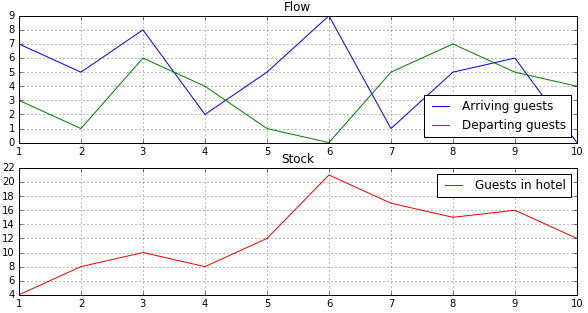# System Dynamics

System Dynamics (or SD as it is often abbreviated) is an instance of equation-based modeling (EBM). It provides a computer-based simulation technique that has been suggested and developed by Jay Wright Forrester in the 1950ies and 60ies. SD is basically a method to conceptualize and calculate dynamics and their interaction in a relatively simple way. It deals with internal feedback loops and time delays that affect the behavior of a system. Its main analytical entities are stocks and flows, which help to understand how seemingly simple systems can display baffling non-linearity.

## Stocks and flows

An intuitive way to visualize the difference indicated with the terms stock and flow provides the bathtub example:Stocks indicate the state of a system at a particular moment in time. Flows characterize the rate of change of the system over time. Speaking about the size of a population for instance, usually refers to its stock, i.e. to its state at time $$t$$, while speaking about births usually refers to the flow (in this case the inflow) indicating the change rate of the stock in the period $$t_1$$ to $$t_2$$.

Differentiating stocks and flows allows a sort of graphical integration. Plotting the flow as net rate of change (= inflow - outflow) as in the plot to the right, visualizes the amount about which the stock has changed as the black area between curve and x-axes. The value of the stock at time $$t_1=S_1$$. Adding the area under the net rate curve between times $$t_1$$ and $$t_2$$ increases the stock to $$S_2$$.Changes in stocks as induced by changes in flows become obvious when plotted beneath each other.### The units of stocks and flows

It is important to understand that flows and stocks are measured differently and thus are incommensurable. While stocks are actual amounts and measured in units – as for example the amount of money on a bank account at a given moment of time – flows are dynamics – as for example the increase of the bank account over a period of time. Flows are measured in units per period.

As mentioned in the chapter on the transition from difference to differential equations, the mathematical abstraction of a differential equation considers these periods to be infinitely small. Therefore, flows often are mistaken for states instead of rates. However, they are dynamics abstracted to a moment in time.

It is important to keep the difference of flows and stocks in mind. When depicted graphically their behavior differs severely, as the following interactive table demonstrates. The left column with plots shows the dynamics of flows, the right column shows the corresponding dynamics of stocks.

Flows $$\frac{dN}{dt}$$

Stocks $$N$$

Note also that the aggregation of stocks is subject to delays in respect to flows. In the following plots the peaks of stocks lag behind the peak of flow about one quarter cycle.The following plots show a stock that increases by the impact of a (more or less) decreasing inflow (blue) and decreases by the impact of an outflow (green). Think of a hotel with people arriving and other people departing.As discussed in the section on exponential growth, the rate of change of a stock - e.g. of an exponentially growing population of size $$N$$ - can be denoted as

$\frac{dN}{dt}=\gamma N$

The rate of change is called a flow in SD . Its counterpart $$N_t$$ is called a stock and indicates the consequences that the flow has on a certain amount of $$N$$ after $$t$$ time steps. Expressed in terms of a difference equation the impact of the flow $$\frac{dN}{dt}$$ on the stock $$N_t$$ would be $$N_{t+1}=N_t+$$$$\gamma N * dt$$

The flow $$\gamma N_t$$ indicates the speed of this dynamic at time t. The size of the stock at time t can be calculated with $$N_t= N_0(1 + \gamma)^t$$ (or $$N_t=N_0e^{\gamma t}$$), which plotted for $$t$$ describes the blue curve in the plot below. In order to find the actual speed in time $$t$$, one needs to find the slope of the curve at time $$t$$. For this, the derivative $$\frac{dN}{dt}$$ of $$N_t= N_0(1 + \gamma)^t$$ is taken, which is $$(1 + \gamma)^t N_0 ln{(1 + \gamma)}$$, and a tangent in $$t$$ is drawn in order to indicate the slope. In general, the tangent of a function$$f(x)$$ at the point $$x=a$$ has the form $$f(a)+f'(a)(x-a)$$$$N_t= N_0(1 + γ)^t$$ with a tangent $$y=N_{50}+N'_{50}(t-50)$$ in $$t = 50$$, for $$0≤t≤100$$

A table for finding derivatives can be accessed here

To make this a bit more practical, consider the speed of a car on a highway cruising from A to B. To find the car's speed at point $$x$$ of this cruise we need to find the slope of the car’s dynamics at $$x$$, which geometrically is the tangent in the point $$x$$ of the curve that plots these dynamics.

The second derivative $$f''$$ of the car's dynamics in $$x$$ would indicate the car's acceleration in point $$x$$.